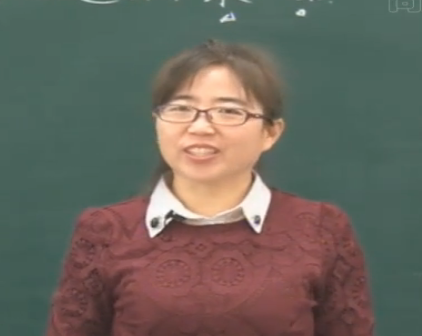尊敬的用户：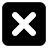三年级数学上册第四章《乘与除》植树—两位数除以一位数的口算

• 同步课程
• 课后作业
• 同步试卷
• 本节重点
• 主讲老师

3×12=  6×15=  13×5=
42×2=  14×7=  21×4=
160÷8=  200÷5=  350÷7=
400÷4=  400÷8=  630÷9=

36÷3=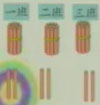30÷3=10
6÷3=2
10+2=12

36÷3=12（棵）

48÷4=12（组）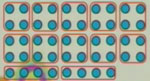40÷4=10
8÷4=2
10+2=12

1.分一分，算一算，说说你是怎么想的。
46÷2=23   39÷3=133.赛跑
46÷1=46  42÷2=21  630÷9=70  84÷4=21
100÷5=20 720÷8=90 86÷2=43   400÷8=50

30÷3=10
+3        +1
33÷3=11
+3        +1
36÷3=12
+3        +1
39÷3=13

20÷2=10
22÷2=11
24÷2=12
26÷2=13

42÷3=14
45÷3=15

40÷4=10
44÷4=11
48÷4=12
52÷4=13

2.手套 鞋子
5元  55元
（1）一双鞋的价钱是一副手套的几倍？
（2）一双鞋比一副手套贵多少钱？
55÷5=11

一、主观题

1．连一连。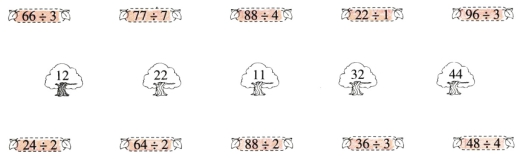【参考答案】：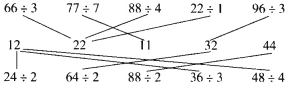2．买文具。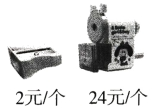(1)手摇削笔机的价格是卷笔刀的多少倍？
(2)（易错题）王老师有50元，买了1个手摇削笔机，剩下的钱能买多少个卷笔刀？

【参考答案】：(1)24÷2=12

(2)（50-24)÷2=13（个）

3．学校举行游泳比赛，三年级一共有48人参加，每4人一组，可以分成多少组？还可以怎样分组？

【参考答案】：48÷4=12（组）

48÷2=24（组）

4．露露从同学那里借来一本148页的故事书，已经看了5天，每天看12页。可是，再过4天就要还给同学了，她应该在剩下的4天里，平均每天比原来多看多少页？【试题解析】：先根据总页数和看了的页数用减法算出还剩下多少页没看，再用除法把剩下的页数平均分成4份，每份就是剩下的4天里每天应该看的页数，最后用剩下的4天里每天看的页数减去原来每天看的页数。

【参考答案】：148-12×5=88（页）  88÷4=22（页）
22-12=10（页）